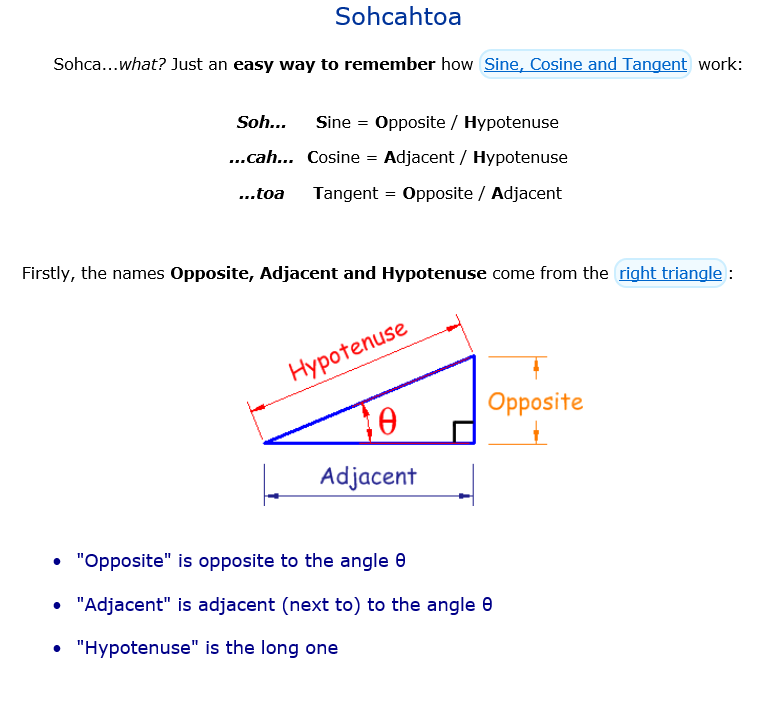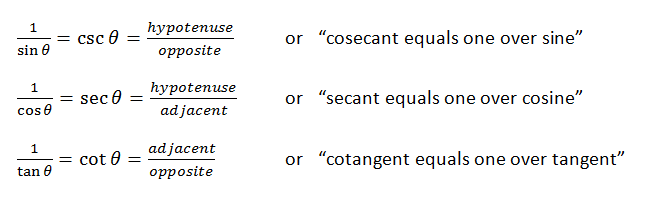# Also Better With Pictures — The Trigonometry Ratios

One of the digressions we follow almost every time I teach AP Physics is intended to give my students a better visual understanding of the 6 basic trig ratios.  But it also resolves several mysteries left over from their first introduction to trig.

When you first learned the trig ratios back in geometry class, you were probably taught the mnemonic SOHCAHTOA.  You can also find this many places on the web.  For example, here is a summary from www.mathisfun.com:Already, we have a bit of a mystery.  Students don’t know where the word “sine” comes from, but that’s fine.  Lots of new topics introduce new vocabulary without going into the etymology of every new term.  But what about “cosine”?  What is co- about it?  A minor mystery to be sure, but even so…

Then we tell them about “tangent”.  We say it is “opposite over adjacent”, which is the TOA in SOHCAHTOA.  And a tiny bit of algebra shows that the tangent can also be written as sine over cosine.  But we still have a mystery:

What is “tangent” about the tangent?

This is not just an etymology question.  The word “tangent” is already part of our math vocabulary.  We saw in geometry that at tangent line is a line that touches but does not cross a circle.  There are all kinds of rules about the angles and the lengths associated with tangent lines (and secant lines too, for that matter).  But what does that use of the word have to do with the way we are using it now, here in trigonometry?

From there, we move on to the reciprocal trig ratios:And now you have more mysteries:

What does this secant have to do with the secant we learned about in geometry?

In geometry class, a secant is a line that crosses a circle.  Is there some reason we are re-purposing this vocabulary?

And as long as we are asking questions:

Why did you match “secant” with “one over COSINE”? And “cosecant”  with “one over SINE”?  Isn’t that making things unnecessarily complicated?

And once again:

What is “co-” about the cosecant?  And the cotangent?

All of these mysteries can be resolved with a single diagram.  I will develop the diagram step-by-step in my next post.  But for now, here it is in the finished form.  Ponder it well…As you will see in the next posts, there are lots of similar triangles waiting to be discovered in this diagram.  Also, the Pythagorean theorem makes several useful appearances.

But for now, let’s resolve one of the mysteries:

Look at the segments labeled sine, secant and tangent.  Note their positions relative to the central angle, θ.

Now look at the segments labeled cosine, cosecant and cotangent.  Note their positions relative to the central angle 90-θ.  Each of these segments relate to 90-θ the way their corresponding segments relate to θ. And remember that 90-θ is called the “complement” of the angle.  So that’s where these segments get their names:

“the complement’s sine” —> cosine

“the complement’s secant” —> cosecant

“the complement’s tangent” —> cotangent

One mystery solved: “co-” is for “complement” !

More mysteries to be unraveled soon.